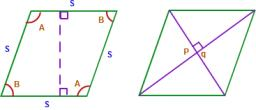# Diagonals of a rhombus

One of the diagonals of a rhombus is twice as the other. If the sum of the lengths of the diagonals is 24, find the area of the rhombus.

Correct result:

S =  64

#### Solution:We would be pleased if you find an error in the word problem, spelling mistakes, or inaccuracies and send it to us. Thank you!Tips to related online calculators
Do you have a linear equation or system of equations and looking for its solution? Or do you have quadratic equation?

#### You need to know the following knowledge to solve this word math problem:

We encourage you to watch this tutorial video on this math problem:

## Next similar math problems:

• Rhombus diagonalArea of rhombus is 224. One diagonal measures 33, find length of other diagonal.
• Rhombus and diagonalsThe lengths of the diamond diagonals are e = 48cm, f = 20cm. Calculate the length of its sides.
• Diamond diagonalsFind the diamond diagonal's lengths if the area is 156 cm2 and side is 13 cm long.
• Two diagonalsThe diagonals of the diamond EFGH have lengths in the ratio 1: 2. What is the circumference of a rhombus if the longer of the diagonals is 8 cm long?
• Diamond diagonalsCalculate the diamonds' diagonals lengths if the diamond area is 156 cm square, and the side length is 13 cm.
• Diagonals of a rhombus 2One diagonal of a rhombus is greater than other by 4 cm . If the area of the rhombus is 96 cm2, find the side of the rhombus.
• Diagonals of diamondFind the area and circumference of the diamond ABCD with 15m and 11m diagonals.
• Rhombus and diagonalsThe a rhombus area is 150 cm2 and the ratio of the diagonals is 3:4. Calculate the length of its height.
• RhombusThe rhombus has diagonal lengths of 4.2cm and 3.4cm. Calculate the length of the sides of the rhombus and its height
• Diagonals of the rhombusHow long are the diagonals e, f in the diamond, if its side is 5 cm long and its area is 20 cm2?
• Rhombus 2Calculate the rhombus area, which has a height v=48 mm and shorter diagonal u = 60 mm long.
• A squareA square with a length of diagonals 12cm give: a) Calculate the area of a square b) rhombus with the same area as the square, has one diagonal with a length of 16 cm. Calculate the length of the other diagonal.
• DiagonalsGiven a rhombus ABCD with a diagonalsl length of 8 cm and 12 cm. Calculate the side length and content of the rhombus.
• DiagonalsA diagonal of a rhombus is 20 cm long. If it's one side is 26 cm find the length of the other diagonal.
• Diamond diagonalsCalculate the diamond's diagonal lengths if its content is 156 cm2 and the side length is 13 cm.
• Rhombus 29One of the diagonals of a rhombus is equal to a side of the rhombus. Find the angles of the rhombus.
• Area of iso-trapFind the area of an isosceles trapezoid if the lengths of its bases are 16 cm and 30 cm, and the diagonals are perpendicular to each other.